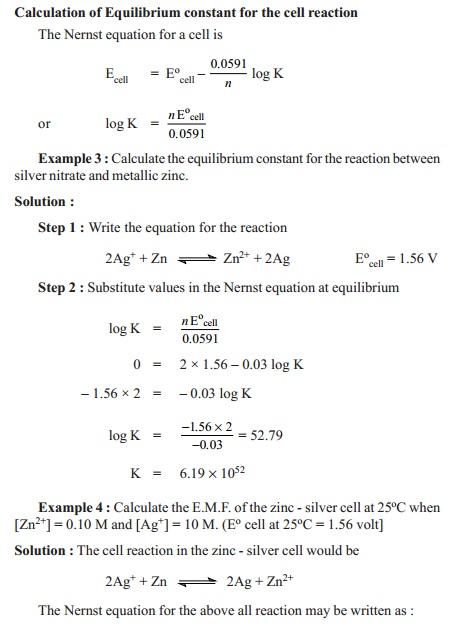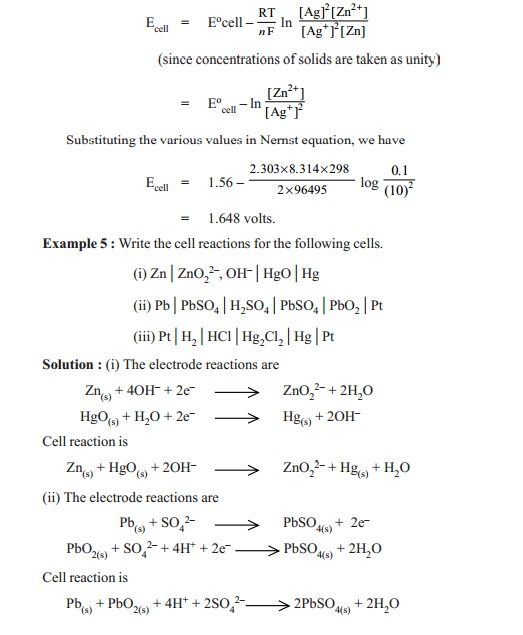Home | Nernst equation - Thermodynamics of a reversible cell

# Nernst equation - Thermodynamics of a reversible cell

Nernst equation : Suppose the reaction occurring in a reversible cell is represented by the equation A + B < -- -- > C + D The decrease in free energy, - DG, accompanying the process is given by the well known thermodynamic equation

Thermodynamics of a reversible cell

Nernst equation : Suppose the reaction occurring in a reversible cell is represented by the equation

A + B < -- -- > C + D

The decrease in free energy, - DG, accompanying the process is given by the well known thermodynamic equation

DG = - D Go  - RT ln J

where - DGo is the decrease in free energy accompanying the same process when all the reactants and products are in their standard states of unit activity and J stands for the reaction quotient of the activities of the products and reactants at any given stage of the reaction.

Substituting the value of J, we have

- D G = - DGo - RT ln (aCxaD /aAxaB )

If E is the E.M.F. of the cell in volts and the cell reaction involves the passage of ' n' faradays (i.e.,) nF coulombs, the electrical work done by the cell is in nFE volt-coulombs or Joules. Hence free energy decrease of the system, - DG, is given by the expression

DG = nFE

nFE = - DGo - RT ln (aCxaD /aAxaB )

nFE = - nFE o - RT ln (aCxaD /aAxaB )

E =Eo  - RT/nF ln (aCxaD /aAxaB )

where Eo is the E.M.F. of the cell in which the activity, or as an approximation, the concentration of each reactant and each product of the cell reaction is equal to unity. Eo is known as the standard E.M.F. of the cell.

E =Eo  - RT/nF ln (aCxaD /aAxaB ) is often referred to as the Nernst equation

Replacing activities by concentrations, as an approximation, the Nernst equation may be written as

E =Eo  - 2.303RT/nF log K

where         Eo  = standard electrode potential

R  = gas constant

T  = Kelvin temperature

n        = number of electrons transferred in the half-reaction

= equilibrium constant for the half-cell reaction as in equilibrium law.

Calculation of Half-cell potential

For an oxidation half-cell reaction when the metal electrode M gives Mn+ ion,

M      -- -- -- >    Mn+ + ne-

the Nernst equation takes the form

E =Eo  - 2.303RT/nF log ( [Mn+] / [M] )

The activity of solid metal [M] is equal to unity. Therefore, the Nernst equation can be written as

E =Eo  - 2.303RT/nF log ( [Mn+]  )

Substituting the values of R, F and T at 25oC, the quantity 2.303 RT/F comes to be 0.0591. Thus the Nernst equation  can be written in its simplified form as

E =Eo  -  0.0591 /n log ( [Mn+])

This is the equation for a half-cell in which oxidation occurs. In case it is a reduction, the sign of E will have to be reversed.

Calculation of Cell potential

The Nernst equation is applicable to cell potentials as well. Thus,

Ecell =Ecello  -  0.0591 /n log ( [Mn+])

K is the equilibrium constant of the redox cell reaction.Study Material, Lecturing Notes, Assignment, Reference, Wiki description explanation, brief detail
11th 12th std standard Class Organic Inorganic Physical Chemistry Higher secondary school College Notes : Nernst equation - Thermodynamics of a reversible cell |